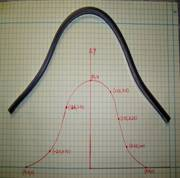## Length of a curve experiment

In a previous post, I mentioned that I have incorporated experiments in my Numerical Methods course. Here I will discuss the second experiment.In this experiment, we find the length of two curves generated from the same points – one curve is a polynomial interpolant and another one is a spline interpolant.

Motivation behind the experiment: In 1901, Runge conducted a numerical experiment to show that higher order interpolation is a bad idea. It was shown that as you use higher order interpolants to approximate f(x)=1/(1+25x2) in [-1,1], the differences between the original function and the interpolants becomes worse. This concept also becomes the basis why we use splines rather than polynomial interpolation to find smooth paths to travel through several discrete points.

What do students do in the lab: A flexible curve (see Figure) of length 12″ made of lead-core construction with graduations in both millimeters and inches is provided. The student needs to draw a curve similar in shape to the Runge’s curve on the provided graphing paper as shown. It just needs to be similar in shape – the student can make the x-domain shorter and the maximum y-value larger or vice-versa. The student just needs to make sure that there is a one-to-one correspondence of values.

Assigned Exercises: Use MATLAB to solve problems (3 thru 6). Use comments, display commands and fprintf statements, sensible variable names and units to explain your work. Staple all the work in the following sequence.

1. Signed typed affidavit sheet.
2. Attach the plot you drew in the class. Choose several points (at least nine – do not need to be symmetric) along the curve, including the end points. Write out the co-ordinates on the graphing paper curve as shown in the figure.
3. Find the polynomial interpolant that curve fits the data. Output the coefficients of the polynomial.
4. Find the cubic spline interpolant that curve fits the data. Just show the work in the mfile.
5. Illustrate and show the individual points, polynomial and cubic spline interpolants on a single plot.
6. Find the length of the two interpolants – the polynomial and the spline interpolant. Calculate the relative difference between the length of each interpolant and the actual length of the flexible curve.
7. In 100-200 words, type out your conclusions using a word processor. Any formulas should be shown using an equation editor. Any sketches need to be drawn using a drawing software such as Word Drawing. Any plots can be imported from MATLAB.

Where to buy the items for the experiment:

1. Flexible curves – I bought these via internet at Art City. The brand name is Alvin Tru-Flex Graduated Flexible Curves. Prices range from $5 to$12. Shipping and handling is extra – approximately $6 plus 6% of the price. You may want to buy several 12″ and 16″ flexible curves. I had to send a query to the vendor when I did not receive them within a couple of weeks. Alternatively, call your local Art Store and see if they have them. 2. Engineering Graph Paper – Staples or Office Depot. Costs about$12 for a pack for 100-sheet pad.
3. Pencil – Anywhere – My favorite store is the 24-hour Wal-Mart Superstore. $1 for a dozen. 4. Scale – Anywhere – My favorite store is the 24-hour Wal-Mart Superstore.$1 per unit.

This post is brought to you by Holistic Numerical Methods: Numerical Methods for the STEM undergraduate at http://nm.mathforcollege.com